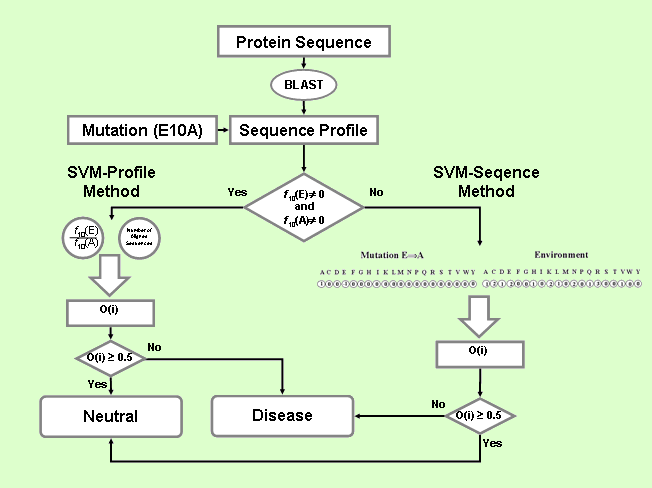PhD-SNP Predictor of human Deleterious Single Nucleotide Polymorphisms
 Last Update 28/08/06

PhD-SNP:  Predictor of human Deleterious Single Nucleotide Polymorphisms

PhD-SNP is based on a decision tree with the SVM-based classifier (SVM-Sequence) coupled to SVM-Profile trained on sequence profile information (see figure below).
PhD-SNP comprises the following steps:

• for a given protein, its sequence profile is built according to the procedure detailed above. From this we evaluate both the frequency of the wild type (fk(wt)) and mutated (fk(mut)) residues at position k. The normalization factor is the number of sequences in the alignment at a given position
• when the frequency of the wild type (fk(wt)) and mutated (fk(mut)) residues at position k are different from 0, the value of fk(wt)/fk(mut) is computed and in conjunction with the total num-ber of aligned sequences in position k is provided to the SVM-Profile method trained on the sequence profile HumVarProf set;
• when no profile is returned at a given position for either wild type or mutated residue, fk(wt)=0 or fk(mut)=0. The prediction is performed with the SVM-Sequence method that was trained on HumVar set, as described below.The SVM-based method using sequence information (SVM-Sequence)
The first SVM classifies mutations into diseases related (desired output set to 0) and neutral polymorphism (desired output set to 1). The decision threshold is set equal to 0.5. The input vector consists of 40 values: the first 20 (the 20 residue types) explicitly define the mutation by setting to -1 the element corresponding to the wild type residue and to 1 the newly intro-duced residue (all the remaining elements are kept equal to 0). The last 20 input values encode for the mutation sequence environment (again the 20 elements represent the 20 residue types). Each input is provided with the number of the encoded residue type, to be found inside a window centered at the residue that undergoes the mutation and that symmetrically spans the sequence to the left (N-terminus) and to the right (C-terminus) with a length of 19 residues [1,2]. For SVM implementation we use LIBSVM (http://www.csie.ntu.edu.tw/~cjlin/) with a RBF kernel function K(xi,xj)=exp(-G ||xi -xj ||2)

The SVM-based method using profile information (SVM-Profile)
The second SVM method (SVM-Profile) classifies mutations into disease and neutral polymorphism taking as input only a vector of 2 elements derived from the sequence profile. This is computed from the output of the BLAST program , running on the nr95 database (E-value threshold=10-9 , number of runs=1) as obtained with cd-hit program available at http://bioinformatics.org/cd-hit/ . The first input element is the ratio between the frequencies of wild-type versus that of the mutated residue in the sequence mutated position and the second element is the number of aligned sequences with respect to the mutation at hand. The software and the kernel used for this SVM implementation are as described above.

Results
In the table some scoring indexes of the efficiency of three methods are listed.

 Q2 P(D) Q(D) P(N) Q(N) C PhD-SNP 0.74 0.80 0.76 0.65 0.70 0.46 SVM-Sequence 0.70 0.71 0.84 0.65 0.46 0.34 SVM-Profile 0.70 0.74 0.49 0.68 0.46 0.39

The overall accuracy Q2 is:

Q2=p/N

where p is the total number of correctly predicted residues and N is the total number of residues.
The correlation coefficient C is defined as:

C(s)=[ p(s)n(s)-u(s)o(s) )] / D

where D is the normalization factor

D =[(p(s)+u(s))(p(s)+o(s))(n(s)+u(s))(n(s)+o(s))]1/2

for each class s (D and N, for disease-related and neutral polymorphism, respectively); p(s) and n(s) are the total number of correct predictions and correctly rejected assignments, respectively, and u(s) and o(s) are the numbers of under and over predictions.

The coverage for each discriminated structure s is evaluated as:

Q(s)=p(s)/[ p(s)+u(s)]

where p(s) and u(s) are as defined above. The probability of correct predictions P(s) (or accuracy for s) is computed as:

P(s)=p(s) / [p(s) + o(s)]

where p(s) and o(s) are previously defined (ranging from 1 to 0).

Required Inputs
PhD-SNP is optimized to predict if a given sinle point protein mutation can be classified as disease-related or as neutral polymorphism. The required inputs are:

• Protein Sequence: the protein sequence can be provided in raw format or giving its Swiss-Prot or uploading a text file containing the protein sequence;
• Position: the position number in the sequence of the residue that undergoes mutation;
• New Residue: if you would ask for a specific mutation please insert the symbol of the mutated residue;
• Prediction: choose between Sequence-Based or Sequence and Profile-Based prediction.
The results can be sent to your e-mail address, if you ask for it, or obtained interactively if you do not past your e-mail in the proper box.

Outputs
The output consists of a table listing the number of the mutated position in the protein sequence, the wild-type residue, the new residue and if the related mutataion is predicted as disease-related (Disease) or as neutral polymorphism (Neutral).
The RI value (Reliability Index) is evaluated from the output of the support vector machine O as

RI=20*abs(O-0.5).

 Capriotti, E., Fariselli, P., Calabrese, R. and Casadio, R. (2005) Predicting protein stability changes from sequences using support vector machines. Bioinformatics, 21 (Suppl 2), ii54-ii58.
 Capriotti, E., Fariselli, P. and Casadio, R. (2005) I-Mutant2.0: predicting stability changes upon mutation from the protein sequence or structure. Nucleic Acids Res., 33 (Web server issue), W306-W310.
 Altschul, S. F., Madden, T. L., Schaffer, A. A., Zhang, J., Zhang, Z., Miller, W. and Lipman, D. J. (1997) Gapped BLAST and PSI-BLAST: a new generation of pro-tein database search programs. Nucleic Acids Res., 25, 3389-3402.
 Li, W., Jaroszewski, L. and Godzik, A. (2001) Clustering of highly homologous sequences to reduce the size of large protein databases. Bioinformatics, 17, 282-283.